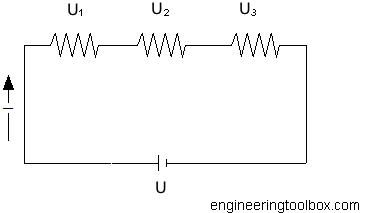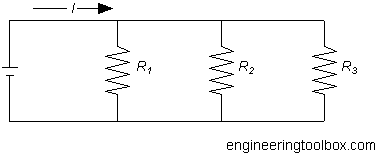Engineering ToolBox - Resources, Tools and Basic Information for Engineering and Design of Technical Applications!

# Electrical Resistance in Serial and Parallel Networks

## Resistors in parallel and serial connections.

### Serial ConnectionThe total resistance for resistors connected in series can be calculated as

R = R1 + R2 + .... + Rn                   (1)

where

R = resistance (ohm, Ω)

#### Example - Resistors in Series

Three resistors 33 ohm, 33 ohm and 47 ohm are connected in serial. The total resistance can be calculated as

R = (33 ohm) + (33 ohm) + (47 ohm)

= 113 ohm

### Parallel ConnectionThe total resistance for resistors connected in parallel can be calculated as

1 / R = 1 / R1 + 1 / R2 + .... + 1 / Rn                  (2)

Equivalent resistance of 2 resistors connected in parallel can be expressed as

R = R1 R2 / (R1 + R2)                    (3)

#### Example - Resistors in Parallel

Three resistors 33 ohm, 33 ohm and 47 ohm are connected in parallel. The total resistance can be calculated as

1 / R = 1 / (33 ohm) + 1 / (33 ohm) + 1 / (47 ohm)

= 0.082 (1 / ohm)

R = 1 / (0.082 ohm)

= 12.2 ohm

If the battery voltage is 12 V - the current through the circuit can be calculated by using Ohm's law

I = U / R

= (12 V) / (12.2 ohm)

= 0.98 ampere

The current through each resistor can be calculated

I1 = U / R1 = (12 V) / (33 ohm) = 0.36 ampere

I2 = U / R2 = (12 V) / (33 ohm) = 0.36 ampere

I3 = U / R3 = (12 V) / (47 ohm) = 0.26 ampere

### Resistors Connected in Parallel - Calculator

Add the resistances for up to five parallel connected resistors and (optionally) the circuit voltage.

The total resistance and current - and the individual currents in all resistors - will be calculated:

R1 (ohms)

R2 (ohms)

R3 (ohms)

R4 (ohms)

R5 (ohms)

Voltage (V)

I1 (amps)

I2 (amps)

I3 (amps)

I4 (amps)

I5 (amps)

R (ohms)

I (amps)

## Related Topics

• Electrical - Electrical units, amps and electrical wiring, wire gauge and AWG, electrical formulas and motors.

## Engineering ToolBox - SketchUp Extension - Online 3D modeling!

Add standard and customized parametric components - like flange beams, lumbers, piping, stairs and more - to your Sketchup model with the Engineering ToolBox - SketchUp Extension - enabled for use with the amazing, fun and free SketchUp Make and SketchUp Pro .Add the Engineering ToolBox extension to your SketchUp from the SketchUp Pro Sketchup Extension Warehouse!

Translate

## Privacy

We don't collect information from our users. Only emails and answers are saved in our archive. Cookies are only used in the browser to improve user experience.

Some of our calculators and applications let you save application data to your local computer. These applications will - due to browser restrictions - send data between your browser and our server. We don't save this data.

## Citation

• Engineering ToolBox, (2014). Electrical Resistance in Serial and Parallel Networks. [online] Available at: https://www.engineeringtoolbox.com/resistance-serial-parallel-connections-d_1881.html [Accessed Day Mo. Year].

Modify access date.

. .

#### Scientific Online Calculator6 24